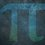# UKMT Specials Note

Here are the links to all my UKMT Special notes:

UKMT Special (Problem $1$)

UKMT Special (Problem $3$)

UKMT Special (Problem $4$)

UKMT Special (Problem $5$)

UKMT Special (Problem $6$)

UKMT Special (Problem $7$)

UKMT Special (Problem $8$)

UKMT Special (Problem $9$)

UKMT Special (Problem $10$)

UKMT Special (Problem $11$)

UKMT Special (Problem $12$)

UKMT Special (Problem $13$)

UKMT Special (Problem $14$)

UKMT Special (Problem $15$)

UKMT Special (Problem $16$)

UKMT Special (Problem $17$)

UKMT Special (Problem $18$)

UKMT Special (Problem $19$)

UKMT Special (Problem $20$)

UKMT Special (Problem $21$)

UKMT Special (Problem $22$)

And in case you want me to continue the series:

UKMT Specials Thoughts

You will have around $4 - 14$ days to solve the special (depending on the difficulty).

Last solution submission time will always be $3:00$pm. On weekdays, I'll post the solution at $4:00$pm, weekends, I'll post the solution at $3:30$pm.

All the problems have been taken from my UKMT database (took me $\frac{3}{2}$ days to complete it!)

If you want the official database, go to their website:

UKMT Solo Competitions

The recent ones are free, but the older ones, you have to buy!

As for commenting, commenting is strictly restricted to my comments or reports - general questions about one of the questions can be posted in the special's chat space.

Update: For BMO solutions, I will be looking through solutions by video on this website:

BMO Solutions Website ($2006$-present)Note by Yajat Shamji
7 months ago

This discussion board is a place to discuss our Daily Challenges and the math and science related to those challenges. Explanations are more than just a solution — they should explain the steps and thinking strategies that you used to obtain the solution. Comments should further the discussion of math and science.

When posting on Brilliant:

• Use the emojis to react to an explanation, whether you're congratulating a job well done , or just really confused .
• Ask specific questions about the challenge or the steps in somebody's explanation. Well-posed questions can add a lot to the discussion, but posting "I don't understand!" doesn't help anyone.
• Try to contribute something new to the discussion, whether it is an extension, generalization or other idea related to the challenge.
• Stay on topic — we're all here to learn more about math and science, not to hear about your favorite get-rich-quick scheme or current world events.

MarkdownAppears as
*italics* or _italics_ italics
**bold** or __bold__ bold
- bulleted- list
• bulleted
• list
1. numbered2. list
1. numbered
2. list
Note: you must add a full line of space before and after lists for them to show up correctly
paragraph 1paragraph 2

paragraph 1

paragraph 2

[example link](https://brilliant.org)example link
> This is a quote
This is a quote
    # I indented these lines
# 4 spaces, and now they show
# up as a code block.

print "hello world"
# I indented these lines
# 4 spaces, and now they show
# up as a code block.

print "hello world"
MathAppears as
Remember to wrap math in $$ ... $$ or $ ... $ to ensure proper formatting.
2 \times 3 $2 \times 3$
2^{34} $2^{34}$
a_{i-1} $a_{i-1}$
\frac{2}{3} $\frac{2}{3}$
\sqrt{2} $\sqrt{2}$
\sum_{i=1}^3 $\sum_{i=1}^3$
\sin \theta $\sin \theta$
\boxed{123} $\boxed{123}$

## Comments

Sort by:

Top Newest

Problem $1$ solving time:

$7$

days

- 7 months ago

Log in to reply

Problem $2$ solving time:

$13$

days

- 7 months ago

Log in to reply

Problem $3$ solving time:

$6$

days

- 7 months ago

Log in to reply

Problem $4$ solving time:

$4$

days

- 7 months ago

Log in to reply

Problem $5$ solving time:

$5$

days

- 7 months ago

Log in to reply

Problem $6$ solving time:

$4$

days

- 7 months ago

Log in to reply

Problem $7$ solving time:

$14$

days

- 7 months ago

Log in to reply

Problem $8$ solving time:

$14$

days

- 7 months ago

Log in to reply

Problem $9$ solving time:

$4$

days

- 7 months ago

Log in to reply

Problem $10$ solving time:

$7$

days

- 7 months ago

Log in to reply

Problem $11$ solving time:

$8$

days

- 7 months ago

Log in to reply

Problem $12$ solving time:

$14$

days

- 7 months ago

Log in to reply

Problem $13$ solving time:

$7$

days

- 7 months ago

Log in to reply

Problem $14$ solving time:

$4$

days

- 7 months ago

Log in to reply

Problem $15$ solving time:

$4$

days

- 7 months ago

Log in to reply

Problem $16$ solving time:

$8$

days

- 7 months ago

Log in to reply

Problem $17$ solving time:

$4$

days

- 7 months ago

Log in to reply

Problem $18$ solving time:

$4$

days

- 7 months ago

Log in to reply

Problem $19$ solving time:

$4$

days

- 7 months ago

Log in to reply

Problem $20$ solving time:

$7$

days

- 7 months ago

Log in to reply

Problem $21$ solving time:

$7$

days

- 7 months ago

Log in to reply

Problem $22$ solving time:

$7$

days

- 7 months ago

Log in to reply

×

Problem Loading...

Note Loading...

Set Loading...